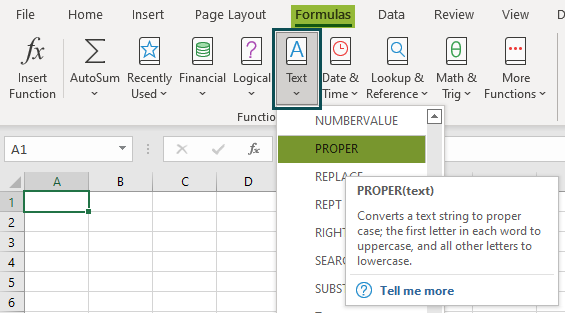# PROPER Excel FunctionArticle byExcelMojo Team## What Is PROPER Function In Excel?

The PROPER Excel function is used when we have a string of text or a sentence that is not in a proper format. The function considers only text as an input. It converts the text into a proper case in which every word’s first letter will be in the upper case, and the rest of the letters will be in lower case.

For example, the image below shows the name in cell A1. But, first, let us apply the PROPER function in Excel to calculate the output.

The steps used to edit the data using the proper function are as follows:

• Select the cell where we want to display the output. In this case, let us select cell B1.
• Enter the PROPER formula, =PROPER(A1), in cell B1.
• Press Enter key.
• The function has returned the result as ‘Kerrie Lacy.’

Likewise, we can use PROPER function in Excel to make the data appear in the right format.

###### Key Takeaways
• The PROPER Excel function is used to capitalize each word in a given string.
• The syntax of the PROPER excel function is =PROPER(text).
• Here, text is the mandatory argument indicating the text or string. The first character in each word will be converted into uppercase and all remaining characters into lowercase.
• The function converts the first letter of the text into uppercase and all remaining characters into lowercase.
• Numbers and punctuation characters remain the same in the PROPER function.

### PROPER() Excel Formula

The Syntax of the PROPER Excel Formula is

where text is the mandatory argument indicating the text or string. The first character in each word will be converted into uppercase and all remaining characters into lowercase.

### How To Use PROPER Excel Function?

We can enable PROPER function with the following two methods.

#### 1.Access from the Excel ribbon

1. Choose the empty cell to display the result.
2. Go to the Formulas tab.
3. Select the Text option from the menu.
4. Select PROPER from the drop-down menu.
5. The Function Arguments window appears.
6. Based on the number of arguments, enter the value in the Text.
7. Select OK.

#### 2. Enter the worksheet manually

1. Select an empty cell to display the output.
2. Type =PROPER( in the selected cell. Alternatively, type =P and double-click the PROPER function from the list of suggestions shown by Excel.
3. Enter the arguments as Excel cell references or direct values.
4. Close the parenthesis and press the Enter key.

Let us take a basic example to understand PROPER Excel function.

The below table shows the string in column A. First, we will reset the text in the string using the PROPER function.

The steps to reset the strings using PROPER Excel function are as follows:

Step 1: First, select the cell where we will enter the formula and calculate the result.

The selected cell, in this case, is cell B2.

Step 2: Then, enter the PROPER Excel function formula in cell B2. The entered value of the text argument is cell A2.

So, the complete formula is =PROPER(A2).

Step 3: Press Enter key.

We can see that the function has returned the text in the right format.

Similarly, we can use the PROPER function to change the data in the right format.

### Examples

#### Example 1

The following table lists the name of stores in column A in incorrect format. Let us reset the data using the PROPER function in Excel.

The steps to reset the strings with PROPER Excel function are as follows:

Step 1: Select the cell where we will enter the formula and calculate the result. The selected cell, in this case, is cell B2.

Step 2: Enter the PROPER() formula in cell B2. The entered value of the text argument is cell A2.

The complete formula is =PROPER(A2).

Step 3: Press Enter key.

The function has displayed the result in cell B2, as shown in the following image.

Step 4: Drag the cursor to cell B5 to obtain the results in the cell range A3:B5.

Likewise, we can use PROPER Excel function to alter the text in the right format.

#### Example 2

The below table shows the format of text in column A and the text string in respective formats in column B, respectively. Now, let us reset the text in the string using the PROPER function.

The steps to reset the strings by PROPER Excel function are as follows:

Step 1: Select the cell where we will enter the formula and calculate the result. The selected cell, in this case, is cell B4.

Step 2: Enter the PROPER() formula in cell B4. The entered value of the text argument can be B2 or B3 since the text is the same in both cells. In our example, let us use cell B2.

The complete formula is =PROPER(B2).

Step 3: Press Enter key.

We can see that the function has returned the result as in cell B4, as shown in the following image.

Similarly, we can use the PROPER function in excel to alter the text in proper format.

#### Example 3

The following table shows the first and last names of people in columns A and B, respectively. Using the PROPER function in Excel, let us reset the name and combine it to form the full name.

The steps to reset the full name by PROPER() in Excel are as follows:

Step 1: Select the cell where we will enter the formula and calculate the result. The selected cell, in this case, is cell C2.

Step 2: Enter the PROPER formula in cell C2. The entered value of the text argument is cell A2 and B2.

So, the complete formula is =PROPER(A2&“ ”&B2).

Step 3: Press Enter key.

The function has returned the result in cell C2, as shown in the following image.

Step 4: Drag the cursor to cell C5 to obtain the result in other cells.

#### Example 4

The following table shows various text strings in column A. But, first, let us reset the strings using the PROPER function in Excel.

The steps to reset the string by PROPER() in Excel are as follows:

Step 1: Select the cell where we will enter the formula and calculate the result. The selected cell, in this case, is cell B2.

Step 2: Enter the PROPER formula in cell B2. The entered value of the text argument is cell A2.

The complete formula is =PROPER(A2).

Step 3: Press Enter key.

The function has returned the result in cell B2, as shown in the following image.

Step 4: Drag the cursor to cell B5 to alter the data in the right format.

Instantly, we can change the data into the right format using the PROPER excel function.

### Important Things To Note

• The PROPER function in Excel does not affect the string, the numbers, and the punctuation characters.
• The PROPER function converts the small case letters to upper case letters and vice versa.
• The function is used when we have a text string to capitalize the first alphabet of each word in the text string and make all the other characters lowercase. This could be the case when we have names in different formats, and we want to make it consistent by capitalizing the first alphabet of the first and last names.

What does the PROPER function do in Excel?

The PROPER function in Excel sets the first letter in a text string as the upper case and any other letters in the text string as the lower case that follow any character other than a letter.

When we import data from external sources in the financial analysis, the PROPER function converts text into the proper case. We use the function to capitalize each word in a given string. The function does not affect numbers and punctuation. The function converts all other letters to lowercase and the first letter in uppercase.

The formula of the PROPER function in Excel is =PROPER(text).

How to insert the PROPER function in Excel?

To insert the PROPER function in Excel, we should follow the below steps;

1. Select the cell to display the output.
2. Type =PROPER( or type =P and double-click the PROPER function from the list of suggestions shown by Excel.
3. Enter the cell reference values.
4. Close the bracket and press the Enter key.

The below image shows text strings in column A. But, first, let us reset the strings using the PROPER function in Excel.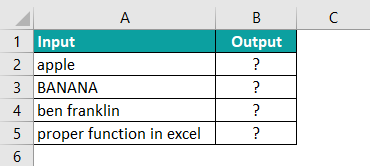The steps to reset the string by the PROPER() in Excel are as follows:

Step 1: Select the cell where we will enter the formula and calculate the result. The selected cell, in this case, is cell B2.

Step 2: Enter the PROPER formula in cell B2. The entered value of the text argument is cell A2.

The complete formula is =PROPER(A2).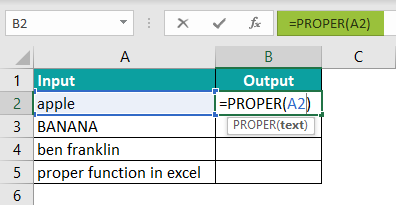Step 3: Press Enter key.

The function has returned the result in cell B2, as shown in the following image.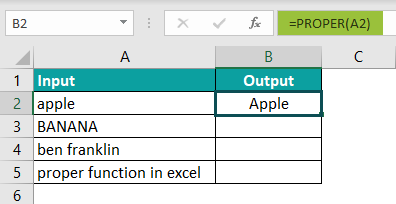Step 4: Drag the cursor to cell B5 to obtain results in other cells.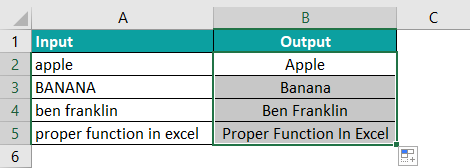Similarly, we can use PROPER excel function to change the format of text.

Where is the PROPER function in Excel?

We can enable PROPER Excel function using the following steps:

1. Choose the empty cell which will contain the result.
2. Go to the Formulas tab.
3. Select the Text group.
4. Select PROPER from the drop-down menu.
5. The Function Arguments window appears.
6. Based on the number of arguments, enter the value in the Text.
7. Select OK.